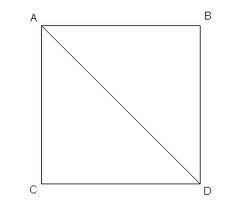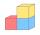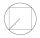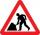# Square diagonal

Calculate the length of diagonal of the square with side a = 11 cm.

d =  15.56 cm

### Step-by-step explanation:Did you find an error or inaccuracy? Feel free to write us. Thank you!Math student
Why do you need to do
d squared = a squared + a squaredDr Math
just  simple Pythagorean theroremTips to related online calculators
Pythagorean theorem is the base for the right triangle calculator.

#### You need to know the following knowledge to solve this word math problem:

We encourage you to watch this tutorial video on this math problem:

## Related math problems and questions:

• Square diagonalCalculate the length of the square diagonal if the perimeter is 172 cm.
• ABCD squareIn the ABCD square, the X point lies on the diagonal AC. The length of the XC is three times the length of the AX segment. Point S is the center of the AB side. The length of the AB side is 1 cm. What is the length of the XS segment?
• DiagonalsGiven a rhombus ABCD with a diagonalsl length of 8 cm and 12 cm. Calculate the side length and content of the rhombus.
• DiagonalThe rectangular ABCD trapeze, whose AD arm is perpendicular to the AB and CD bases, has an area of 15 cm square. Bases have lengths AB = 6cm, CD = 4cm. Calculate the length of the AC diagonal.
• Diagonal of squareCalculate the side of a square when its diagonal is 10 cm.
• A squareA square with a length of diagonals 12cm give: a) Calculate the area of a square b) rhombus with the same area as the square, has one diagonal with a length of 16 cm. Calculate the length of the other diagonal.
• CalculateCalculate the length of the wall diagonal of the cube with an edge 5 cm long.
• CalculateCalculate the length of a side of the equilateral triangle with an area of 50cm2.
• Find theFind the length of the side of the square ABCD, which is described by a circle k with a radius of 10 cm.
• Squares above sidesTwo squares are constructed on two sides of the ABC triangle. The square area above the BC side is 25 cm2. The height vc to the side AB is 3 cm long. The heel P of height vc divides the AB side in a 2: 1 ratio. The AC side is longer than the BC side. Calc
• Diamond diagonalsCalculate the diamonds' diagonals lengths if the diamond area is 156 cm square, and the side length is 13 cm.
• Triangle in a squareIn a square ABCD with side a = 6 cm, point E is the center of side AB, and point F is the center of side BC. Calculate the size of all angles of the triangle DEF and the lengths of its sides.
• Rectangle diagonalThe rectangle, one side of which is 5 cm long, is divided by a 13 cm diagonal into two triangles. Calculate the area of one of these triangles in cm2.
• Two diagonalsThe rhombus has a side length of 12 cm and a length of one diagonal of 21 cm. What is the length of the second diagonal?
• Diagonal - simpleCalculate the length of the diagonal of a rectangle with dimensions 5 cm and 12 cm.
• Side and diagonalFind the circumference and the area of the rectangle if given: side a = 8 cm diagonal u = 10 cm.
• IS trapezoidCalculate the length of diagonal u and height v of isosceles trapezoid ABCD, whose bases have lengths a = |AB| = 37 cm, c = |CD| = 29 cm and legs b = d = |BC| = |AD| = 28 cm.